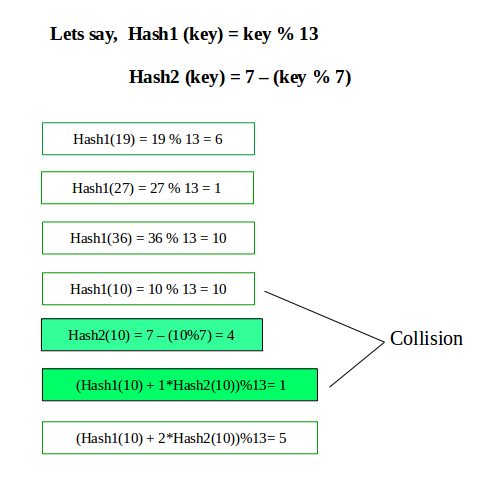# Double Hashing

Double hashing is a collision resolving technique in Open Addressed Hash tables. Double hashing uses the idea of applying a second hash function to key when a collision occurs.

Double hashing can be done using :
(hash1(key) + i * hash2(key)) % TABLE_SIZE
Here hash1() and hash2() are hash functions and TABLE_SIZE
is size of hash table.
(We repeat by increasing i when collision occurs)

Attention reader! Don’t stop learning now. Get hold of all the important DSA concepts with the DSA Self Paced Course at a student-friendly price and become industry ready.  To complete your preparation from learning a language to DS Algo and many more,  please refer Complete Interview Preparation Course.

In case you wish to attend live classes with experts, please refer DSA Live Classes for Working Professionals and Competitive Programming Live for Students.

First hash function is typically hash1(key) = key % TABLE_SIZE

A popular second hash function is : hash2(key) = PRIME – (key % PRIME) where PRIME is a prime smaller than the TABLE_SIZE.

A good second Hash function is:

• It must never evaluate to zero
• Must make sure that all cells can be probed`// CPP program to implement double hashing``#include ``using` `namespace` `std;`` ` `// Hash table size``#define TABLE_SIZE 13`` ` `// Used in second hash function.``#define PRIME 7`` ` `class` `DoubleHash {``    ``// Pointer to an array containing buckets``    ``int``* hashTable;``    ``int` `curr_size;`` ` `public``:``    ``// function to check if hash table is full``    ``bool` `isFull()``    ``{`` ` `        ``// if hash size reaches maximum size``        ``return` `(curr_size == TABLE_SIZE);``    ``}`` ` `    ``// function to calculate first hash``    ``int` `hash1(``int` `key)``    ``{``        ``return` `(key % TABLE_SIZE);``    ``}`` ` `    ``// function to calculate second hash``    ``int` `hash2(``int` `key)``    ``{``        ``return` `(PRIME - (key % PRIME));``    ``}`` ` `    ``DoubleHash()``    ``{``        ``hashTable = ``new` `int``[TABLE_SIZE];``        ``curr_size = 0;``        ``for` `(``int` `i = 0; i < TABLE_SIZE; i++)``            ``hashTable[i] = -1;``    ``}`` ` `    ``// function to insert key into hash table``    ``void` `insertHash(``int` `key)``    ``{``        ``// if hash table is full``        ``if` `(isFull())``            ``return``;`` ` `        ``// get index from first hash``        ``int` `index = hash1(key);`` ` `        ``// if collision occurs``        ``if` `(hashTable[index] != -1) {``            ``// get index2 from second hash``            ``int` `index2 = hash2(key);``            ``int` `i = 1;``            ``while` `(1) {``                ``// get newIndex``                ``int` `newIndex = (index + i * index2) % TABLE_SIZE;`` ` `                ``// if no collision occurs, store``                ``// the key``                ``if` `(hashTable[newIndex] == -1) {``                    ``hashTable[newIndex] = key;``                    ``break``;``                ``}``                ``i++;``            ``}``        ``}`` ` `        ``// if no collision occurs``        ``else``            ``hashTable[index] = key;``        ``curr_size++;``    ``}`` ` `    ``// function to search key in hash table``    ``void` `search(``int` `key)``    ``{``        ``int` `index1 = hash1(key);``        ``int` `index2 = hash2(key);``        ``int` `i = 0;``        ``while` `(hashTable[(index1 + i * index2) % TABLE_SIZE] != key) {``            ``if` `(hashTable[(index1 + i * index2) % TABLE_SIZE] == -1) {``                ``cout << key << ``" does not exist"` `<< endl;``                ``return``;``            ``}``            ``i++;``        ``}``        ``cout << key << ``" found"` `<< endl;``    ``}`` ` `    ``// function to display the hash table``    ``void` `displayHash()``    ``{``        ``for` `(``int` `i = 0; i < TABLE_SIZE; i++) {``            ``if` `(hashTable[i] != -1)``                ``cout << i << ``" --> "``                     ``<< hashTable[i] << endl;``            ``else``                ``cout << i << endl;``        ``}``    ``}``};`` ` `// Driver's code``int` `main()``{``    ``int` `a[] = { 19, 27, 36, 10, 64 };``    ``int` `n = ``sizeof``(a) / ``sizeof``(a);`` ` `    ``// inserting keys into hash table``    ``DoubleHash h;``    ``for` `(``int` `i = 0; i < n; i++) {``        ``h.insertHash(a[i]);``    ``}``    ``// searching some keys``    ``h.search(36); ``// This key is present in hash table``    ``h.search(100); ``// This key does not exist in hash table``    ``// display the hash Table``    ``h.displayHash();``    ``return` `0;``}`
Output:
```36 found
100 does not exist
0
1 --> 27
2
3
4
5 --> 10
6 --> 19
7
8
9
10 --> 36
11
12 --> 64
```

My Personal Notes arrow_drop_up